# Curved Line - Definition with Examples

The Complete K-5 Math Learning Program Built for Your Child

• 40 Million Kids

Loved by kids and parent worldwide

• 50,000 Schools

Trusted by teachers across schools

• Comprehensive Curriculum

Aligned to Common Core

## Curved Line

Imagine an ant has to move from point A to point B. What are the different ways in which the ant can reach from point A to point B?The ant can take several paths to reach from point A to B. The given figures show some of the paths that the ant can take to reach from point A to point B.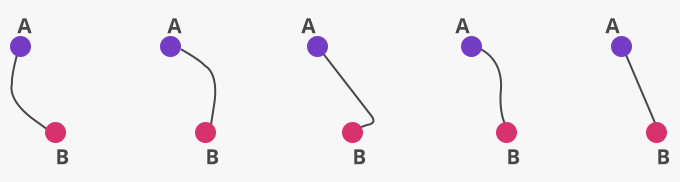We see that in the first four figures, the ant changed its direction while traveling from point A to point B, that is, it did not follow one constant direction. However, in the last figure, the ant moved straight, and the distance it moved was the shortest. The movement from one point to another gives rise to straight or curved lines.

 Straight Line Curved Line The shortest line joining any 2 points is a straight line. If a point moves in only one direction, we get a straight line.A line that is not straight is a curved line.  If a point does not move in one direction, we get a curve.## Examples of the curved line

The above letters and numbers are made of only curves.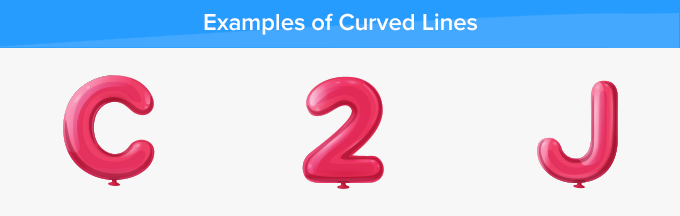Non-examples of the curved line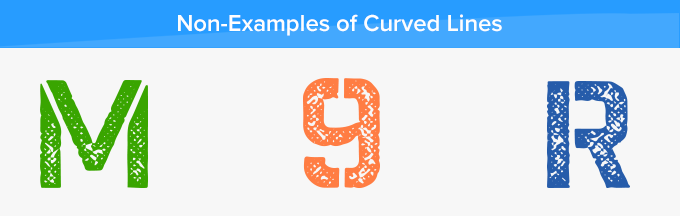The above letters and numbers are made by joining straight lines.

Curved lines form open and closed curves.

Open curves: The examples of open curves are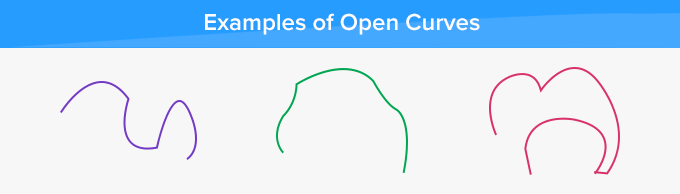Closed Curves: The examples of closed curves are.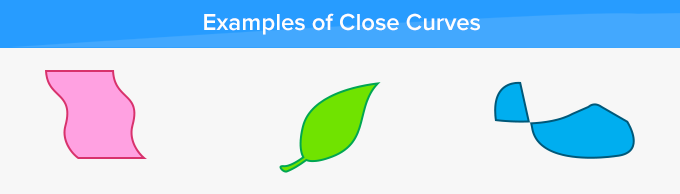Circle: A circle is a closed curve formed when a point moves in a plane such that it is at a constant distance from its center.Fun Fact Geometry, a branch of mathematics, deals with different figures and solids made up of straight and curved lines.

Won Numerous Awards & Honors